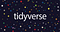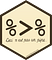# Into the tidyverse: dplyr

## The dplyr package offers alternatives to base R functions.

I completed an introductory course in using R for statistical analysis. The instructions were in the underlying base functions in R (base R).

This article compares the code for data manipulation, using dplyr and base R. This article only covers basic tasks.

# Data management and manipulation

Introductory courses ask students to undertake simple manipulate of data frames. These courses may use the base R functions.

Since 2016, there have been a collection of packages which share the same design philosophy. As the packages focus on ‘tidy’ data, designers call this the tidyverse.Let’s begin. (Image: tidyverse.org)

In this article, I will use the standard iris data-set in R.

One basic step is to count how many rows meet one criterion. We want to know how many plants in our data-set are in the Setosa species.

In base R, we turn the data frame into a series of ‘TRUE’ and ‘FALSE’ entries. When we sum, that turns ‘TRUE’ values into 1, and ‘FALSE’ into 0. The sum then counts the rows which meet the criterion.

`sum(iris\$Species == "setosa")`

In dplyr, we often use the ‘forward-pipe’ operator `%>%`. This operator will forward a value or resulting expression into the next expression. That way, we can build our query — step by step.This is not a pipe. (Image: magrittr)
`iris %>%  filter(Species == "setosa") %>%  count()`

Instead of a single count, we can produce frequency tables. In base R, that uses the length function on the Species column.

`tapply(iris\$Species, iris\$Species, length)`

In the tidyverse, we count by species:

`iris %>% count(Species)`

Another common manipulation is to create a subset. This is similar in base R and tidyverse code.

`iris_setosa_df <- subset(iris, iris\$Species == "setosa")iris_setosa_df <- iris %>% filter(Species == "setosa")`

We may wish to take the top rows of the data set. In base R, the order function puts the vector elements in a certain order. That order is then used on the data set. The head function takes the top six rows:

`head(iris_setosa_df[order(iris_setosa_df\$Petal.Length, decreasing = TRUE), ], n = 6)`

In tidyverse, we use arrange and desc to arrange the rows in descending order (as the names suggest). The slice_head function to take the first six rows:

`iris_setosa_df %>%  arrange(desc(Petal.Length)) %>%  slice_head(n = 6)`

We often want to create new variables. There are two different ways of doing this in base R:

`iris_setosa_df\$sepal_ratio <- iris_setosa_df\$Sepal.Length / iris_setosa_df\$Sepal.Widthiris_setosa_df[["sepal_ratio"]] <- iris_setosa_df[["Sepal.Length"]] / iris_setosa_df[["Sepal.Width"]]`

For this purpose, we can use the mutate function in the tidyverse:

`iris_setosa_df <- iris_setosa_df %>%  mutate(sepal_ratio = Sepal.Length / Sepal.Width)`

The tidyverse functions may offer a plainer way to learn for people new to R.

The R code is available on GitHub and R Pubs.

This blog looks at the use of statistics in Britain and beyond. It is written by RSS Statistical Ambassador and Chartered Statistician @anthonybmasters.

## More from Anthony B. Masters

This blog looks at the use of statistics in Britain and beyond. It is written by RSS Statistical Ambassador and Chartered Statistician @anthonybmasters.